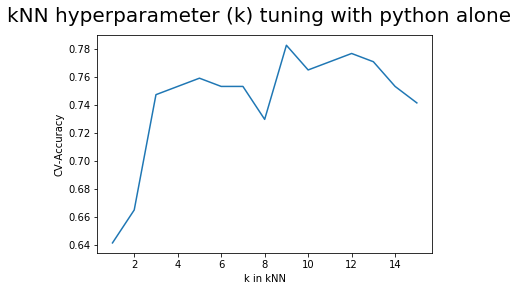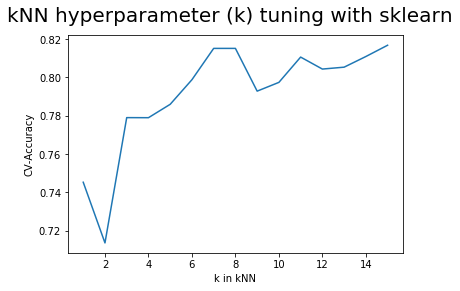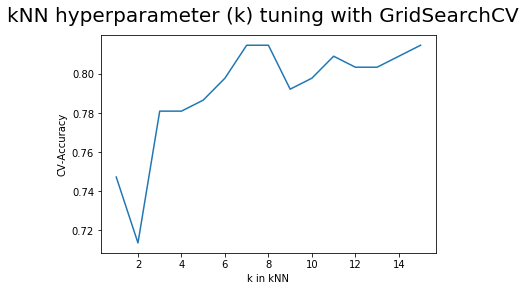In this post, we will go through an approach to get optimal/tuned model for the final prediction. First, we will see how to select best 'k' in kNN using simple python example. We will then jump to using sklearn apis to explore different options for hyperparameter tuning.

For previous post, you can follow:

How kNN works ?

K-Nearest Neighbors Algorithm using Python and Scikit-Learn?

Out of sample accuracy estimation using cv in knn

In :
import os
import math
from collections import Counter
import numpy as np
import pandas as pd
import matplotlib.pyplot as plt
import warnings
warnings.filterwarnings('ignore')

%matplotlib inline

# making results reproducible
np.random.seed(42)

In :
df = pd.read_csv(

df.columns = ['CLASS', 'ALCOHOL_LEVEL', 'MALIC_ACID', 'ASH', 'ALCALINITY','MAGNESIUM', 'PHENOLS',
'FLAVANOIDS', 'NON_FLAVANOID_PHENOL', 'PROANTHOCYANINS', 'COLOR_INTENSITY',
'HUE', 'OD280/OD315_DILUTED','PROLINE']

# Let us use only two features : 'ALCOHOL_LEVEL', 'MALIC_ACID' for this problem
df = df[['CLASS', 'ALCOHOL_LEVEL', 'MALIC_ACID']]

Out:
CLASS ALCOHOL_LEVEL MALIC_ACID
0 1 14.23 1.71
1 1 13.20 1.78
2 1 13.16 2.36
3 1 14.37 1.95
4 1 13.24 2.59

#### 1. kNN and Cross validation using Python from Scratch¶

In :
class KNN:
def __init__(self, K):
self.K = K
self.X_train = None
self.y_train = None

def fit(self, X_train, y_train):
self.X_train = X_train
self.y_train = y_train

def predict_instance(self, test_instance):
inputs = self.X_train.copy()
# calculate L2 norm between all training points and given test_point
inputs['distance'] = np.linalg.norm(inputs.values-test_instance.values, axis=1)

# concatenate inputs and labels before sorting the distances
inputs = pd.concat([inputs, self.y_train], axis=1)

# sort based on distance
inputs = inputs.sort_values('distance', ascending=True)

# pick k neighbors

# get list from dataframe column
classes = neighbors['CLASS'].tolist()

# create counter of labels
majority_count = Counter(classes)

return majority_count.most_common(1).pop()

def predict(self, X_test):
predictions = np.zeros(X_test.shape)
# we want out index to be start from 0
X_test.reset_index(drop=True, inplace=True)
for index, row in X_test.iterrows():
predictions[index] = self.predict_instance(row)
return predictions

def cross_validation(n, k, data, n_neighbors):
"""
n : # iterations
k : k-fold size
data: training data
n_neighbors: k in knn
"""
accuracies = []

for _ in range(0, n):
# data shuffle
data.sample(frac=1)

fold=int(data.shape/k)

for j in range(k):
test = data[j*fold:j*fold+fold]
train = data[~data.index.isin(test.index)]
X_train, y_train = train.drop('CLASS', axis=1), train['CLASS']
X_test, y_test = test.drop('CLASS', axis=1), test['CLASS']

knn = KNN(n_neighbors)
knn.fit(X_train, y_train)

predictions = knn.predict(X_test)
true_values = y_test.to_numpy()
accuracy = np.mean(predictions == true_values)

accuracies.append(accuracy)
return np.array(accuracies).mean()

In :
# We will be using following settings for all the cases below
k_values = np.arange(1, 16)
cross_validation_fold = 10
accuracies = []


#### 2. Finding optimal k value for kNN¶

In :
for k in k_values:
# run cross-validation with given neighbor size k
accuracy = cross_validation(1, cross_validation_fold, df, k)
accuracies.append(accuracy)
print(accuracies)

[0.6411764705882353, 0.6647058823529411, 0.7470588235294118, 0.7529411764705881, 0.7588235294117647, 0.7529411764705882, 0.7529411764705881, 0.7294117647058822, 0.7823529411764707, 0.7647058823529412, 0.7705882352941177, 0.7764705882352941, 0.7705882352941176, 0.7529411764705881, 0.7411764705882352]

In :
fig = plt.figure()
plt.plot(k_values, accuracies)
plt.xlabel('k in kNN')
plt.ylabel('CV-Accuracy')
fig.suptitle('kNN hyperparameter (k) tuning with python alone', fontsize=20)

Out:
Text(0.5, 0.98, 'kNN hyperparameter (k) tuning with python alone')We can see that k=9 seems a good choice for our dataset.

#### 3. Finding optimal k value for kNN using sklearn¶

In :
from sklearn.neighbors import KNeighborsClassifier
from sklearn.model_selection import cross_val_score

In :
# I wanted to see how many cpus are available in my machine so that i can run cv in parallel
os.cpu_count()

Out:
8
In :
X, y = df.drop('CLASS', axis=1), df['CLASS']
accuracies = []
for k in k_values:
# instantiate kNN with given neighbor size k
knn = KNeighborsClassifier(n_neighbors=k)
# run cross validation for a given kNN setup
# I have setup n_jobs=-1 to use all cpus in my env.
scores = cross_val_score(knn, X, y, cv=cross_validation_fold, scoring='accuracy', n_jobs=-1)
accuracies.append(scores.mean())
print(accuracies)

[0.7452936876504987, 0.7136416408668731, 0.7789946680426557, 0.778953818369453, 0.7859391124871001, 0.798765909872721, 0.8151057791537667, 0.8151057791537667, 0.7928083075335397, 0.7973770209838321, 0.8105714654282765, 0.8043214654282765, 0.8053083075335398, 0.8108638630890953, 0.8167462160302718]

In :
fig2 = plt.figure()
plt.plot(k_values, accuracies)
plt.xlabel('k in kNN')
plt.ylabel('CV-Accuracy')
fig2.suptitle('kNN hyperparameter (k) tuning with sklearn', fontsize=20)

Out:
Text(0.5, 0.98, 'kNN hyperparameter (k) tuning with sklearn')Usually, we have to deal with many hyperparameters for a model. In order to tune all of them at once, sklearn has provided a different API. Next, we will explore GridSearchCV.

#### 4. Tune many hyperparameters together using sklearn GridSearchCV API¶

We will be using distance metrices and k-neigbors for this case.
In :
from sklearn.model_selection import GridSearchCV

In :
metrics = ['euclidean','manhattan']
neighbors = np.arange(1, 16)
param_grid  = dict(metric=metrics, n_neighbors=neighbors)
param_grid

Out:
{'metric': ['euclidean', 'manhattan'],
'n_neighbors': array([ 1,  2,  3,  4,  5,  6,  7,  8,  9, 10, 11, 12, 13, 14, 15])}
In :
# here 10-fold cross-validation is being executed for all the combinations
# total combinations will be : 15*2 = 30
# so in total 30 10-fold cross validatin will be run
knn = KNeighborsClassifier()
# when refit=True, it will fits the best hyperparameters to all training data
# and also allow to use GridSearchCV object as an estimator for prediction
grid_search = GridSearchCV(knn, param_grid, cv=cross_validation_fold, scoring='accuracy', refit=True)
grid_search.fit(X, y)

/home/lenovo/workspace/envs/envml/lib/python3.5/site-packages/sklearn/model_selection/_search.py:813: DeprecationWarning: The default of the iid parameter will change from True to False in version 0.22 and will be removed in 0.24. This will change numeric results when test-set sizes are unequal.
DeprecationWarning)

Out:
GridSearchCV(cv=10, error_score='raise-deprecating',
estimator=KNeighborsClassifier(algorithm='auto', leaf_size=30,
metric='minkowski',
metric_params=None, n_jobs=None,
n_neighbors=5, p=2,
weights='uniform'),
iid='warn', n_jobs=None,
param_grid={'metric': ['euclidean', 'manhattan'],
'n_neighbors': array([ 1,  2,  3,  4,  5,  6,  7,  8,  9, 10, 11, 12, 13, 14, 15])},
pre_dispatch='2*n_jobs', refit=True, return_train_score=False,
scoring='accuracy', verbose=0)
In :
cross_val_df = pd.DataFrame(grid_search.cv_results_)

Out:
mean_fit_time mean_score_time mean_test_score param_metric param_n_neighbors params rank_test_score split0_test_score split1_test_score split2_test_score split3_test_score split4_test_score split5_test_score split6_test_score split7_test_score split8_test_score split9_test_score std_fit_time std_score_time std_test_score
0 0.002142 0.002116 0.747191 euclidean 1 {'n_neighbors': 1, 'metric': 'euclidean'} 28 0.736842 0.666667 0.833333 0.666667 0.777778 0.833333 0.777778 0.833333 0.764706 0.5625 0.000315 0.000213 0.082817
1 0.002033 0.002119 0.713483 euclidean 2 {'n_neighbors': 2, 'metric': 'euclidean'} 30 0.684211 0.611111 0.777778 0.666667 0.722222 0.777778 0.611111 0.833333 0.764706 0.6875 0.000164 0.000213 0.070995
2 0.001908 0.001944 0.780899 euclidean 3 {'n_neighbors': 3, 'metric': 'euclidean'} 22 0.736842 0.722222 0.888889 0.722222 0.833333 0.833333 0.888889 0.833333 0.705882 0.6250 0.000115 0.000084 0.082573
3 0.001834 0.001916 0.780899 euclidean 4 {'n_neighbors': 4, 'metric': 'euclidean'} 22 0.736842 0.666667 0.833333 0.722222 0.888889 0.833333 0.888889 0.833333 0.823529 0.5625 0.000063 0.000051 0.097615
4 0.001839 0.001939 0.786517 euclidean 5 {'n_neighbors': 5, 'metric': 'euclidean'} 21 0.736842 0.722222 0.888889 0.722222 0.888889 0.888889 0.777778 0.777778 0.705882 0.7500 0.000107 0.000093 0.070958
In :
# since for both metric (manhatton/euclidean), we will have test score
# let's use euclidean for this case
accuracies = cross_val_df[cross_val_df["param_metric"]=='euclidean']["mean_test_score"]
accuracies

Out:
0     0.747191
1     0.713483
2     0.780899
3     0.780899
4     0.786517
5     0.797753
6     0.814607
7     0.814607
8     0.792135
9     0.797753
10    0.808989
11    0.803371
12    0.803371
13    0.808989
14    0.814607
Name: mean_test_score, dtype: float64
In :
fig3 = plt.figure()
plt.plot(k_values, accuracies)
plt.xlabel('k in kNN')
plt.ylabel('CV-Accuracy')
fig3.suptitle('kNN hyperparameter (k) tuning with GridSearchCV', fontsize=20)

Out:
Text(0.5, 0.98, 'kNN hyperparameter (k) tuning with GridSearchCV')#### 5. Following the standard Machine Learning pipeline¶

• Until now, we are using all the data as our training set
• Within cross validation, data is sampled for training set and also used for building validation set from this sample
• Final validation score is used as a performance measure.
• Now, we will also take out some data for the final testing (this data is not allowed to touch until last testing phase)
In :
from sklearn.model_selection import train_test_split

In :
# Split the dataset in two equal parts
X_train, X_test, y_train, y_test = train_test_split(
X, y, test_size=0.5, random_state=0)

In :
# TRAINING PHASE
knn = KNeighborsClassifier()
grid_search = GridSearchCV(knn, param_grid, cv=cross_validation_fold, scoring='accuracy', refit=True)
grid_search.fit(X_train, y_train)

/home/lenovo/workspace/envs/envml/lib/python3.5/site-packages/sklearn/model_selection/_search.py:813: DeprecationWarning: The default of the iid parameter will change from True to False in version 0.22 and will be removed in 0.24. This will change numeric results when test-set sizes are unequal.
DeprecationWarning)

Out:
GridSearchCV(cv=10, error_score='raise-deprecating',
estimator=KNeighborsClassifier(algorithm='auto', leaf_size=30,
metric='minkowski',
metric_params=None, n_jobs=None,
n_neighbors=5, p=2,
weights='uniform'),
iid='warn', n_jobs=None,
param_grid={'metric': ['euclidean', 'manhattan'],
'n_neighbors': array([ 1,  2,  3,  4,  5,  6,  7,  8,  9, 10, 11, 12, 13, 14, 15])},
pre_dispatch='2*n_jobs', refit=True, return_train_score=False,
scoring='accuracy', verbose=0)
In :
# since refit=True,we can directly use grid_search object above as our final best model or you can do as follow:
optimal_knn = grid_search.best_estimator_
optimal_knn

Out:
KNeighborsClassifier(algorithm='auto', leaf_size=30, metric='euclidean',
metric_params=None, n_jobs=None, n_neighbors=9, p=2,
weights='uniform')
###### You can see that the optimal_knn has neighbor size = 9 and metric = 'euclidean'.¶
In :
# TESTING PHASE
# accuracy on test data
optimal_knn.score(X_test, y_test)

Out:
0.797752808988764
In [ ]: